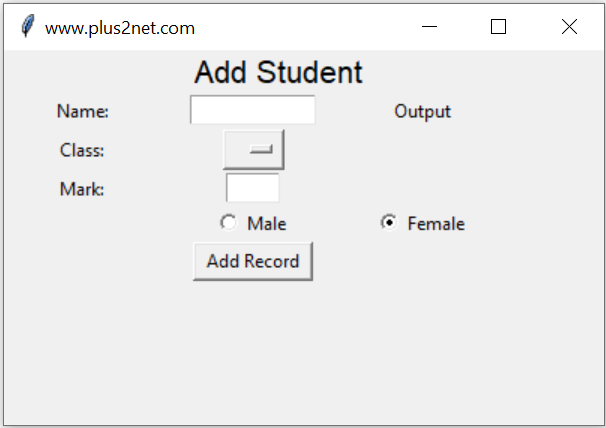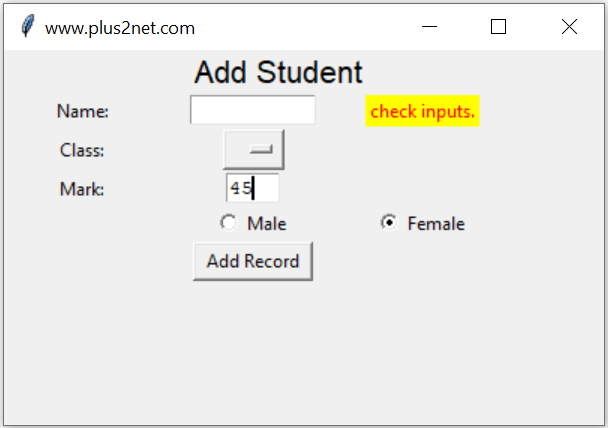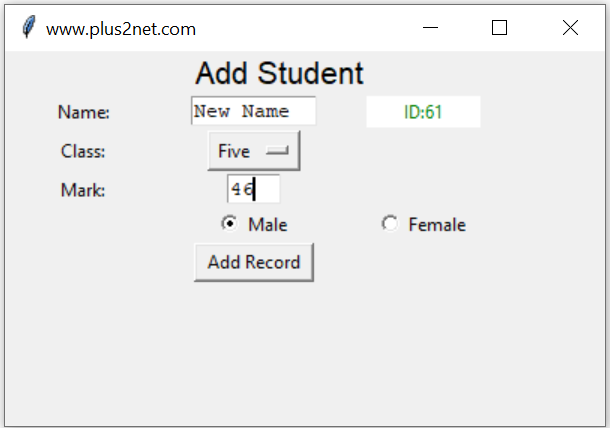# Inserting Data to Excel workbook

Add rows to Excel workbook by taking user entered inputs from a Tkinter window.There are three parts in this script.
1. Part 1: Create the GUI window using tkinter.
2. Part 2: Validate the user entered data.
3. Part 3: Add data to Excel file.

Data input to Excel workbook from Tkinter window by using openpyxl

## Part 1 : Tkinter window to accept user inputs

User will enter four input data using our Tkinter window. Main components are here

Name : String of name through a text box t1
Class : Select one option from the dropdown OptionMenu
Mark : Integer value ( number ) through text box t3
gender : Selection through a pair of radio buttons ( Male , Female or other )
Submit : Button to trigger a click event to handle all the inputs.

## Managing Excel file by openpyxl

We will use openpyxl to manage Excel file.

Some line of code are commented and description is added to explain the reasons. If you want to use one new excel file then include the proper lines.
``````#from openpyxl import load_workbook
path = "E:\data\student7.xlsx"  # existing file to work ( Not a new file. )
To work with a new Excel file.
``````from openpyxl import Workbook
wb = Workbook()  # create workbook

ws = wb.active  # work on default worksheet
#ws = wb.create_sheet("my_student")  # create a worksheet``````
After inserting data, we will save the file ( workbook) .
``````        l1 = [my_name, my_class, my_mark, my_gender] # List to add
ws.append(l1) # data added at the end
wb.save(path)``````

There are Labels to show message about the input data ( l1,l2,l3 ) and one final Label ( l5 ) to display the output or return id of the record after inserting the data in table. One OptionMenu ( opt1) is used for selection of class.
``````l0 = tk.Label(my_w, text="Add Student", font=16, width=30, anchor="c")
l0.grid(row=1, column=1, columnspan=4)

l1 = tk.Label(my_w, text="Name: ", width=10, anchor="c")
l1.grid(row=3, column=1)

t1 = tk.Text(my_w, height=1, width=10, bg="white")
t1.grid(row=3, column=2)

l2 = tk.Label(my_w, text="Class: ", width=10)
l2.grid(row=4, column=1)

# add list box for selection of class
options = StringVar(my_w)
options.set("")  # default value

opt1 = OptionMenu(my_w, options, "Three", "Four", "Five")

l3 = tk.Label(my_w, text="Mark: ", width=10)
l3.grid(row=5, column=1)

t3 = tk.Text(my_w, height=1, width=4, bg="white")
t3.grid(row=5, column=2)

r2.grid(row=6, column=2)

r3.grid(row=6, column=3)

b1 = tk.Button(my_w, text="Add Record", width=10,
b1.grid(row=7, column=2)
my_str = tk.StringVar()
l5 = tk.Label(my_w, textvariable=my_str, width=10)
l5.grid(row=3, column=3)
my_str.set("Output")``````
The Button b1 is used to execute the function add_data() by using the command or click event of the button.
``````b1 = tk.Button(my_w,  text='Add Record', width=10,
b1.grid(row=7,column=2)``````
Inside the function add_data() we have two sections. In first section we will check each input data. It is expected that no input is left blank by user. Without filling the details if user has clicked the button ( b1 ) then we will display a message through our Label ( l5 ) asking to check the inputs ( Read the else part of the if-else).
``````def add_data():
flag_validation=True # set the flag

# length of my_name , my_class and my_gender more than 2
if(len(my_name) < 2 or len(my_class)<2  or len(my_gender) < 2 ):
flag_validation=False
try:
val = int(my_mark) # checking mark as integer
except:
flag_validation=False

if(flag_validation):
#  Part 3  Insert to Excel file
else:
l5.config(fg='red')   # foreground color
l5.config(bg='yellow') # background color
my_str.set("check inputs.")``````In this code we first set one flag validation ( flag_validation ) to True, if any where the validation of the inputs fails then we will change this status of validation to False. ( this is part of the above code )
``````if(len(my_name) < 2 or len(my_class)<2  or len(my_gender) < 2 ):
flag_validation=False
try:
val = int(my_mark) # checking mark as integer
except:
flag_validation=False ``````
After all the checks ( validation of inputs ) if the flag ( flag_validation ) is True , then we can insert data to Excel file and save the file.
``````    if flag_validation:
l1 = [my_name, my_class, my_mark, my_gender]
ws.append(l1)
wb.save(path)
try:
print(" Data to excel ")

except Exception as e:
print(e)``````

Here there is no auto incremented id column like we used in our MySQL database. We can create one incremental number and use that to store in our excel file.We have used the Label l5 to display the id of the record added. This display of Id ensures that the record is inserted successfully. By using ws.max_row we can get the last row number.
``````    if flag_validation:
i = ws.max_row +1 # Next row number
#print(str(ws.max_row) + ", i value :"  + str(i))
l1 = [i, my_name, my_class, my_mark, my_gender]
ws.append(l1)

wb.save(path)``````

We want to show the ID ( after adding the record ) or the error message for some time ( say 3 seconds ) and it should vanish after the time delay.
``l5.after(3000,lambda:l5.config(fg='white',bg='white',text=''))``
For a new file if you are adding incremental row number system then note that first row number is 1 so the ws.max_row will return 1 for first time. So for the first record it will add number 2 as row number as you are incrementing the same by 1.
One if condition is used to prevent the first row number as 2

Remove the user entered text after adding of records. Code to add data row including the reset , time dealy, and the getting the last row number is here.
``````if flag_validation:
try:
#i=int(ws.cell(ws.max_row, 1).value or 0)+1
i=ws.max_row # read the last row number
if i!=1:
i=i+1
#print(str(ws.max_row) + ", i value :"  + str(i))
l1 = [i, my_name, my_class, my_mark, my_gender] # row of data to add
ws.append(l1)

wb.save(path)
t1.delete("1.0", END)  # reset the text entry box
t3.delete("1.0", END)  # reset the text entry box
l5.grid()
l5.config(fg="green")  # foreground color
l5.config(bg="white")  # background color
# my_str.set("ID:" + str(id.lastrowid)) # this is for MySQL only
my_str.set("ID: " + str(ws.max_row))
l5.after(3000, lambda: l5.config(fg="white", bg="white", text=""))``````
``````import tkinter as tk
from tkinter import *

path = "E:\data\student7.xlsx"  # existing file to work ( Not a new file. )

from openpyxl import Workbook
wb = Workbook()  # create workbook

ws = wb.active  # work on default worksheet
#ws = wb.create_sheet("my_student")  # create a worksheet

my_w = tk.Tk()
my_w.geometry("400x250")
my_w.title("www.plus2net.com")

l0 = tk.Label(my_w, text="Add Student", font=16, width=30, anchor="c")
l0.grid(row=1, column=1, columnspan=4)

l1 = tk.Label(my_w, text="Name: ", width=10, anchor="c")
l1.grid(row=3, column=1)

t1 = tk.Text(my_w, height=1, width=10, bg="white")
t1.grid(row=3, column=2)

l2 = tk.Label(my_w, text="Class: ", width=10)
l2.grid(row=4, column=1)

# add list box for selection of class
options = StringVar(my_w)
options.set("")  # default value

opt1 = OptionMenu(my_w, options, "Three", "Four", "Five")

l3 = tk.Label(my_w, text="Mark: ", width=10)
l3.grid(row=5, column=1)

t3 = tk.Text(my_w, height=1, width=4, bg="white")
t3.grid(row=5, column=2)

r2.grid(row=6, column=2)

r3.grid(row=6, column=3)

b1.grid(row=7, column=2)
my_str = tk.StringVar()
l5 = tk.Label(my_w, textvariable=my_str, width=10)
l5.grid(row=3, column=3)
my_str.set("Output")

flag_validation = True  # set the flag
my_name = t1.get("1.0", END)  # read name
my_class = options.get()  # read class
my_mark = t3.get("1.0", END)  # read mark

# length of my_name , my_class and my_gender more than 2
if len(my_name) < 2 or len(my_class) < 2 or len(my_gender) < 2:
flag_validation = False
try:
val = int(my_mark)  # checking mark as integer
except:
flag_validation = False

if flag_validation:
try:
#i=int(ws.cell(ws.max_row, 1).value or 0)+1
i=ws.max_row # read the last row number
if i!=1:
i=i+1
#print(str(ws.max_row) + ", i value :"  + str(i))
l1 = [i, my_name, my_class, my_mark, my_gender] # row of data to add
ws.append(l1)

wb.save(path)
t1.delete("1.0", END)  # reset the text entry box
t3.delete("1.0", END)  # reset the text entry box
l5.grid()
l5.config(fg="green")  # foreground color
l5.config(bg="white")  # background color
# my_str.set("ID:" + str(id.lastrowid)) # this is for MySQL only
my_str.set("ID: " + str(ws.max_row))
l5.after(3000, lambda: l5.config(fg="white", bg="white", text=""))
except Exception as e:
print(e)

else:
l5.grid()
l5.config(fg="red")  # foreground color
l5.config(bg="yellow")  # background color
my_str.set("check inputs.")
l5.after(3000, lambda: l5.config(fg="white", bg="white", text=""))
my_w.mainloop()``````

Subscribe to our YouTube Channel here

## Subscribe

* indicates required
Subscribe to plus2netplus2net.com